DeutschEnglishEspañolFrançaisItalianoMagyarNederlands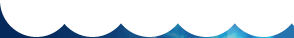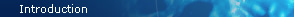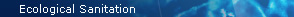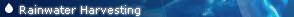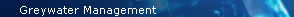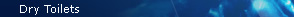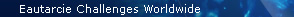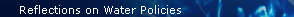Rainwater Harvesting for Whole-House ReuseRainwater QualityWater for General Household UseBiocompatible WaterAbout Hydrogen Water / Alkaline Water (or « Kangen » Water)The meaning of ORPElectrical charge exchanges in waterORP’s first ambiguityUnderlying significance of the rH2 ConceptORP’s second ambiguityVincent’s Quantization value (W)Chlorine and UVImplementing the PLUVALOR SystemCistern Use and MaintenanceFiltration SystemsThe PLUVALOR System on the MarketSearch a word within the website eautarcie.org

To understand this chapter, you need to have a basic knowledge in general chemistry, at college or university level. It will help not only in understanding the true scope of the different processes of water electrolysis, but also the concept of biocompatible water. Still, those who don’t have a scientific background can disregard the mathematical and chemical formulae while still comprehending the basic thinking behind the ideas.

The concept of rH2 is unfortunately not taught in schools, nor is it in most university faculties. And yet, ignorance of this concept can mislead even specialists, when observing and evaluating phenomena that are tied to electron e- and proton H+ transfers.

In the field of medicine, this key concept helps understand and explain the emergence of a number of serious diseases, and chart new avenues of research for the treatment of these diseases.

Let’s not forget that virtually all chemical reactions that drive living beings are reactions of proton transfer (acid-base) and electron transfer (redox). The unitary theory of these two types of reactions gives a fair idea of the intimate interrelationships between these two types of reactions. These interdependencies help understand the mistakes caused when only considering acid-base reactions (pH concept) to explain certain health anomalies (such as « acidification of the body »), regardless of redox reactions.

This chapter is a condensed summary of two scientific articles by József Országh published in the journal Sciences du vivant (Éditions Arys, Paris):

Réactions oxydo-réduction et acido-basiques: Vers une approche théorique et expérimentale plus cohérente (Redox and Acid-Base Reactions: Towards a More Coherent Theoretical and Experimental Approach). Volume 1, pages 23-34 (1990)
L'eau en tant que système redox (Water – as a redox system). Volume 2, pages 81-97 (1991)

The text within this page was first published in French on www.eautarcie.org:
2011-03-01

The original text has been adapted and translated in English by André Leguerrier and was first published on this page: 2011-03-18

Last update: 2015-10-17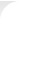# Unitary Theory of Redox and Acid-base Reactions – the Meaning of ORP## Electrical charge exchanges in water

If water and the aqueous medium are considered a « miracle of nature », it is in thanks to their remarkable properties related to the exchange of positive electric charges or H+ protons and negative electric charges or e electrons. The former are the acid-base reactions, the second those of oxidation-reduction. In all the treaties of chemistry, both types of reactions are treated in separate chapters. And yet, with regard to simple transfer of electric charges, these two types of reactions can be described by a single mathematical formalism, the unitary theory of charge transfer reactions in aqueous media. As we will show, this theory clearly shows the intimate connection of these two types of reactions. The considerations herein are obviously only valid in an aqueous medium .


General note: contrary to popular belief, the dissolution of substances in water is not a simple mixing of water molecules and the dissolved substances. In the overwhelming majority of cases, dissolution involves a profound change in the dissolved substances. For example by dissolving table salt – sodium chloride (NaCl) – in water, there is what chemists call electrolytic dissociation, through a true chemical reaction: NaCl (solid) → Na+ (dissolved) + Cl (dissolved). Substances that dissociate into ions (such as the sodium ion Na+ or the chloride ion Cl) in water are electrolytes. Thus in a soup, in reality, there is no longer any salt (as sodium chloride); there remain Na+ and Cl ions. Ions carrying a positive electric charge are cations attracted to a metal electrode called « cathode » bearing negative electrical charges. Similarly, ions carrying a negative electric charge are anions attracted to the anode carrying positive charges.

The main peculiarity of water compared to other solvents is that it actively participates in the reactions that take place in solution. The water acts as a base with respect to acids introduced into it, and as an acid with respect to dissolved alkalis. Similarly, it acts as an oxidant with respect to reducing agents (electron donor) and as a reductant with respect to oxidizing agents (electron acceptor). Moreover, as will be shown, a reaction between an acid (proton donor) and a base (proton acceptor) does not occur directly, but occurs by means of water. A base will pick up the acid’s H+ proton, and a water molecule located nearby will yield another proton to the base. This is how an acid-base neutralisation reaction takes place in water. Similarly, the dissolution of a reductant (electron donor, such as vitamin C) will yield electrons e to water. An oxidant (electron acceptor) will pick up an electron from another water molecule. This is the redox reaction, which constitutes a sort of neutralisation. Therefore the dissolution of an oxidant, a reductant, an acid or a base profoundly modifies the chemical properties of water – and consequently – its biological properties. Water that contains reducing agents (electron donors) will be somehow « enriched » with electrons. In this case, we say that the water is an antioxidant.

#### Auto-protolysis of water

Between two water molecules, there may be spontaneous proton transfer, a positive electric charge (the proton is the nucleus of a hydrogen atom, designated by H+), through a reaction called « auto-protolysis »:

H2O + H2O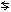H3O+ + OH
(1)

To simplify, chemists often adopt a convention which replaces the writing of the hydroxonium ion H3O+ (in fact the hydrolysed proton, not to be confused with the « hydranion » ion H3O) by the proton H+, knowing of course that this is only a writing convention. In reality, a proton can never « travel » alone in water without being bonded to a molecule of H2O, no more so than hydranion. Therefore, reaction (1) simply reads:

H2OH+ + OH
(1a)

The OH ion is called hydroxide. The equilibrium constant Kw of the auto-protolysis reaction (1a) reads:

Kw = [H+][OH] = 10–14
(2)

...where [H+] and [OH] are the activities of those protons and hydroxide ions. As a first approximation [H+] and [OH] can often be treated as molar concentrations expressed in moles/litre. In electrochemistry and thermodynamics, it is more correct to speak of activity instead of concentration. Activity is a dimensionless number.

Once dissolved in water, acids release protons thereby increasing the activity of H+ ions (which we shall now read as [H+]), called proton activity. On the other hand, the bases are likely to release hydroxide ions OH by increasing their activity [OH]. However, by means of Equation (2) in an aqueous medium, a balance is always established between these two ions, so that the product of their activities remains constant at a value of 10–14. Thus, there is a certain « balance » between the two: when proton activity increases, hydroxide ion activity decreases, and vice versa.

To characterize the acidity or alkalinity of an aqueous solution, Sørensen, a Danish doctor and biochemist introduced in 1909 the concept of « hydrogen potential », which he expressed as « pH2 » ., which later became « pH » that we now define as: pH = – log[H+]. This also calls for the complementary concept of pOH, defined in a similar manner: pOH = –log[OH]. Similarly, the auto-protolysis constant can also be expressed in the same logarithmic form: pKw = –logKw = –log10–14 = 14. Therefore, Equation (2) can be written in a logarithmic form:

pKw = pH + pOH = 14
(3)

Obviously, the reference state for aqueous solutions is chemically pure water in which the acidity and alkalinity are balanced: the medium is neutral. In this case, the activities of protons and hydroxide ions are the same: [H+] = [OH] = 10–7. In a solution where this condition is fulfilled, the result is pH= – log10–7 = 7. This is the point of acid-base neutrality. Solutions with a pH between 0 and 7 are called « acid », those whose pH is between 7 and 14 are called « basic » (or « alkaline ») .


Unwittingly, Sørensen’s pH2 prefigured the notion of rH2 launched in 1920 by the American physical chemist WM Clark. In fact the two variables are closely linked to the H2 hydrogen molecule. Upon contact with water, this molecule undergoes a bona fide electrolytic dissociation: H2 + 2H2OH3O+ + H3O- detected by measuring the potential of the solution in which is sparged hydrogen gas. Sørensen’s pH is none other than -log[H3O+], while Clark’s rH2 is -log[H3O-]. The parallel between the two concepts is more than obvious. Furthermore, neither Sørensen nor Clark were electrochemists. Only, Sørensen was luckier to have friends among the electrochemists of his time unlike Clark who was subjected to a genuine scientific inquisition by the American Chemical Society, for his rH2 concept. In 1924 he was forced to make a public self-criticism and withdraw his invention. Because of this, the science of electrochemistry was deprived of an essential tool, the consequences of which are becoming extremely annoying as they are an obstacle to the development of promising applications in electrochemistry and thermodynamics, particularly in medicine.

Solutions whose pH is less than zero (thus with a negative pH) are called « super acid ». In such solutions, the activity of protons is greater than one. Solutions with a pH above 14, in which the activity of hydroxide ions is greater than one are called « super basic ». In biology and medicine, such aqueous media are not encountered.

#### Auto-electrolysis of water

The concepts outlined in the preceding paragraph are, in principle, known to high school students. Those that follow are far less known to the public, even by specialists.

In the fields of electrochemistry and thermodynamics of aqueous solutions, the reference state is chemically pure water. The standard reaction of formation of water

H2 + ½ O2H2O
(4)

...is the basis for calculating the thermodynamic functions of the water molecule starting from electrochemical and calorimetric data. The reverse of this reaction is what we call « auto-electrolysis » of water that takes place when 4 (negatively charged) electrons e- are exchanged between two water molecules, giving rise to two hydrogen molecules and one oxygen molecule:

H2O + H2O2 H2 + O2
(5)

Reminder: auto-protolysis takes place by (positively charged) proton exchanges between two water molecules. The parallelism between auto-protolysis and auto-electrolysis is obvious. Also see the table in the next chapter. The equilibrium constant Ke for reaction (5) thus reads:

Ke = [H2]2[O2] = 10–84
(6)

In fact, the water molecule’s oxygen yields two electrons to the two hydrogen atoms. These two atoms combine to make a di-hydrogen molecule H2. The oxygen having lost two electrons « seeks » another, in the same situation, to form the di-oxygen molecule O2 . The water's auto-electrolysis constant Ke can be calculated from the standard Gibbs energy (i.e. free enthalpy) of water formation.


This reaction is quite marginal in water, to such an extent that on average, one molecule of di-hydrogen forms in 20,000 litres of water. This has prompted chemists to say that the activity of hydrogen [H2] in water « has no physical significance » since there is no hydrogen in water. They also argued on the extremely low equilibrium constant Ke of auto-electrolysis. Applying the same reasoning, this would have meant« throwing out » a good part of the Tables of solubility product constants for sparingly soluble salts. For example, the solubility product of mercuric sulphide (HgS) is 10–53, that of mercury selenide (HgSe) is 10–59. It is obvious that in a solution « saturated » in these salts, there are no mercury ions, sulphide ions or selenide ions. The solubility product values were calculated from the redox potential (ORP) measured in these solutions. The procedure is exactly the same to calculate an aqueous solution’s rH2.

When dissolving a reducing agent in water, it will tend to yield electrons to the water. On the other hand, the oxidizing agent will capture electrons. The presence of a reducing agent will move the auto-electrolytic balance towards the formation of hydrogen H2, while the oxidizing agent will foster the appearance of oxygen O2. Here, too, thanks to the the equilibrium (5), there is balance between hydrogen [H2] and oxygen [O2] activities. Both interchange, as in the case of [H+] et [OH].

#### Definition of rH2

In 1920, seven years after the pH concept was introduced to the world, W.M. Clark defined a similarly-structured notion , the concept of rH2:

rH2 = –log[H2]
(7)

In parallel, we can also define the rO2 = –log[O2]. Thenceforth, considering Equation (6), Equation (3) for redox reactions will read:

pKe = 2 rH2 + rO2 = 84
(8)

In 1920, Clark called this concept « rH ». The rH2 symbol - which is more correct - only appeared in the 1940’s. Clark had defined the rH value as « the negative logarithm of hydrogen pressure in Water »: rH = –logPH2. At the time, the concept of thermodynamic activity was not yet known. Clark's error was to assert that « the rH is the measure of the oxidative or reductive strength of a dissolved substance in water », which is obviously false. Clark's contemporaries did not understand the underlying genius of this invention which was in fact the indispensable complement to Sørensen’s pH concept. After heated discussions within the American Chemical Society, Clark eventually bowed to pressure from his colleagues. In 1924, he acknowledged his « mistake » and advised his contemporaries to abandon the use of rH. Despite this self-criticism, given its practicality, the use of rH spread to laboratories around the world. It took until 1954 before this fundamental concept was set aside. Source: W.M. Clark, « The Determination of Hydrogen Ions », Vol.2, Plenum New York (1920).

#### « Redox neutrality » or the concept of « redox indifference »

For redox reactions, the reference medium is also chemically pure water, in which hydrogen [H2] and oxygen [O2] activities are divided in proportions of one oxygen for two hydrogen: [H2] = 2 [O2]. Let’s therefore calculate the rH2 of chemically pure water. To do so, in Equation (6), replace [O2] by ½[H2]

Ke = ½ [H2]3 = 10–84

...from which one obtains the activity of hydrogen in pure water: [H2] = 10–27.7 that chemists round to 10–28. Pure water’s rH2 is therefore –log10–28 = 28. This is called the point of « redox neutrality » or « redox indifference ».

So regardless of the pH value, an aqueous solution is neither oxidative nor reductive (or anti-oxidative) when its rH2 is equal to 28. A solution whose rH2 is located between 0 and 28 is called reductive and antioxidant. When the value of rH2 is between 28 and 42, the solution is called oxidant .


In biology, an aqueous medium whose rH2 is below 28 is called « anaerobic ». Indeed, in such an environment, oxygen [O2] activity is very low. Bacteria that may develop there have a chemically reductive action. A typical example is the presence of azobacter cultures capable of reducing nitrate ions NO3- into atmospheric nitrogen N2 in an anaerobic milieu. In wastewater treatment, this is called « anaerobic denitrification ».

Once the rH2 rises above 28, we are in « aerobiosis ». The oxygen activity here being higher, other types of bacteria come to develop. In fact, each species of bacterium, virus or fungus has a well-defined pH and rH2 in which it can develop. Outside their pH and rH2 range, these microscopic creatures die. In a Cartesian coordinate system, we can carry the rH2 values with respect to pH for define a two-dimensional space, in which the domain of existence of each bacterium or virus can be delineated. This gives a map of viral and bacterial activity in which each species occupies a definite place. Such a diagram is called a « Vincent Diagram »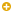.

We can now understand that even modest changes to blood’s rH2 have a decisive influence on the possible development or disappearance of a given bacterium or virus. Medical applications of electrochemistry here open promising perspectives. The use of hydrogen water is just one example. In fact, the value of blood’s rH2 also has a decisive influence on the electrical potentials that are established within cells between the nucleus and cytoplasm, and between the cytoplasm and intercellular fluids. These potentials also regulate cell division, and thus the potential genesis of cancer cells.

#### Stability limits of aqueous solutions

A solution in which the activity of oxygen [O2] = 1, rO2 being zero under Equation (8), rH2=42. This is the upper limit of thermodynamic stability of an aqueous solution. At this value, gaseous oxygen O2 is released, but especially, highly reactive atomic oxygen O is formed: what used to be called « nascent oxygen ».

A solution in which rH2 is zero has an activity of hydrogen [H2] = 1. It is the lower limit of thermodynamic stability of an aqueous solution. At this value, water decomposes and releases « nascent hydrogen » H, electrically neutral. This hydrogen has a high affinity to free radicals, which are also carriers of an unpaired electron.

A negative value for the rH2 has in principle no physical meaning. However, negative values have been measured under particular conditions. Here we enter the realm of reversible processes in the field of thermodynamics. We are looking at aqueous solutions that are unstable from a thermodynamic perspective, in which nascent hydrogen is temporarily stabilised in a different molecular formation: hydranion H3O. This is probably the case of hydrogen water.

## ORP’s first ambiguity

Potential redox ORP is an experimental measure (in volts or millivolts) widely used to characterize the redox properties of aqueous solutions. It is generally accepted that the greater the ORP, the more oxidative the solution; the lower the ORP, the more the solution is reductive or « anti-oxidative ». This assertion is only true at pH = constant.

The ORP readings on a measuring instrument have two ambiguities:

• It’s not the actual ORP used in electrochemical and thermodynamic calculations. For this, the reading must be corrected.
• Even the real (i.e. corrected) ORP is only one of the elements needed ascertain the oxidative or antioxidative character of an aqueous solution.

Now let's look at the first ambiguity.

#### Conventional scale of ORP potentials

Now consider the nature of ORP from an electrochemical point of view. The ORP scale’s true zero point does not correspond to the zero potential reading observed on a voltmeter. The latter in no way represents a solution’s redox point of indifference (or neutrality), i.e. above which the solution is oxidative, and below which it is reductive. In fact, the zero potential stems from the concept of thermodynamic stability limits in aqueous solutions. This condition is achieved at the standard hydrogen electrode, more specifically a platinum electrode coated with a metallic platinum sponge (also called a « platinized » platinum electrode), dipped in a solution having a pH=0, in which hydrogen gas H2 is sparged at a pressure of one bar.

The hydrogen adsorbed in the pores of the platinum sponge behaves as an element in a condensed state, in which the thermodynamic activity is equal to: [H2] = 1. So the electrolyte in contact with the platinum electrode has an rH2 of zero. At pH = rH2 = 0, on the platinum electrode, we measure a remarkably stable potential with a value is zero {under the Nernst Law: Equation (11)} . The thermodynamic zero potential therefore has a precise physical meaning; its value is linked to the simultaneous cancellation of pH and rH2.


In current treaties of electrochemistry, the only condition mentioned about the standard hydrogen electrode is that pH=0. The condition of « 1 bar or 1 atm. hydrogen pressure » from which [H2] = 1, is somewhat confusing. Indeed, under this pressure, hydrogen’s solubility in water is only about one thousandth of a mole/litre. In this concentration range, the dissolved hydrogen content can, in first approximation, be equated with the activity: [H2]. By linking the measured potential with the condition of 1 bar hydrogen pressure, a conceptual mistake is made. The electrolyte that’s in contact with hydrogen and the platinum electrode has an rH2 value that is actually not zero, but about 3.

This assertion is easily verified experimentally. By replacing the « platinized » platinum electrode by a bright platinum electrode, even under a hydrogen pressure of 1 bar and a pH = 0, the measured potential stabilizes between +85 and +90 mV instead of 0 mV. The reason is that the rH2 in the bright platinum electrode is not zero, but about –log10–3 = 3, despite the hydrogen pressure of 1 bar. To bring rH2 back to zero, the hydrogen in contact with the platinum should be in a condensed state: only this state can have a thermodynamic activity equal to one. With a platinized platinum electrode, hydrogen gas « condenses » in the cavities of the platinum sponge thereby reducing rH2 to zero. It is therefore simpler and more consistent to define the standard hydrogen electrode as a platinum electrode in which the pH = rH2 = 0.

To dispel misunderstandings leading to errors, remember that the zero potential reading on an ORP measuring instrument does not equate to the thermodynamic ORP and in no way corresponds to the redox indifference point above which there is an oxidative milieu, and below which there is a reductive or (antioxidant) milieu.

#### Measuring the ORP

To measure a solution’s ORP, two electrodes are plunged into it: a platinum electrode (inert vs water) and a reference electrode with a stable potential (inert vs the solution) . The resulting difference in potential between the two electrodes is thence measured, not the ORP. In the overwhelming majority of cases, the reference electrode is a silver chloride electrode (Ag/AgCl) whose potential is +200 millivolts (+0.2 volts) with respect to the standard hydrogen electrode (which is 0 Volt by definition). The platinum electrode thus takes the solution’s potential ORP, but the voltmeter displays the difference in potential between the ORP and the reference electrode’s potential. It is therefore quite inappropriate to designate the potential displayed by the voltmeter as being the « ORP ».


Modern electrodes that are used to measure the ORP are so-called « combined » electrodes in which a reference electrode Ag/AgCl is placed next to the platinum electrode, as in your typical commercial ORP electrode. The ORP of this battery’s electrolyte is what’s measured. So, you don’t measure a « potential », but rather the electromotive force (emf) of a conventional battery.

To compare, a solution’s pH is also measured using a « combined » electrode. Here too, you measure the emf of a battery composed of a silver/silver-chloride reference electrode and a glass electrode. The latter’s potential is proportional to the logarithm of proton activity: pH = –log[H+].

There is no technical or conceptual difference between pH and of rH2 measurements. These two quantities are complementary and precisely define the state of protonation and electronification of an aqueous solution. Ignoring rH2 makes it difficult, even impossible, to determine a solution’s oxidative state with precision.

## Underlying significance of the rH2 concept

#### What is « electron activity »?

Everyone agrees that pH expresses the proton exchange level in an aqueous solution. However, without an understanding of rH2, scientists incorrectly consider that the oxidative or reductive properties of a solution are characterized only by the ORP. We will now show that this is true only under special circumstances(only at pH = constant). In most cases, the assumption is wrong.

The paragraphs on water’s auto-protolysis and auto-electrolysis have shown that only hydrogen [H2] activity can characterize a solution’s oxidative or reductive state with respect to the reference state (i.e. chemically pure water). Hydrogen [H2] activity is in fact the solution’s electron activity, since H2 is formed by the transfer of two electrons e on two water protons H+. Thus the activity [H2] expresses the solution’s state of electronicization. But we can then ask what the measured ORP really means. This brings us to ORP’s second ambiguity.

## ORP’s second ambiguity

The classical Nernst equation (equation 11) explicitly defines the ORP by relating it to activities of the oxidized [Ox] and reduced [Red] forms of a given redox (couple) system. Each system is characterized by a redox reductive half-reaction: Oxidative + n electronsReductive. A system’s oxidized form thus captures n electrons to transform into its reduced form. By convention, chemists write this process using an equation:

Oxidant + n electronsReductant

The oxidized form of a system thus captures n electrons to turn into its reduced form. By convention, chemists write the process using the following equation:

Ox + n eRed
(9)

For example, the chloride/chlorine system or Cl/Cl2 is characterized by the half-reduction reaction: Cl2 + 2 e2 Cl. Oxidative chlorine Cl2 thus captures two electrons e, and is thereby reduced into chloride ions Cl. Similarly, the sodium ion Na+ (which is simply the oxidized form of metallic sodium Na) can also capture an electron to be reduced into metallic sodium Na, as per the reaction Na+ + e → Na.

A major fact is that water, as an active solvent, has an amphoteric (or amphiprotic) character, i.e. it behaves as an acid vis-à-vis a base, and as a basis vis-à-vis an acid. The same goes for oxidants and reductants: in the presence of a reducing agent, water behaves as an oxidant (it captures electrons), whereas in the presence of an oxidant, it acts as a reductant by yielding electrons.

So water is itself a redox system that can give or capture electrons from the substances that are dissolved into it. Water’s redox system is the hydrogen/proton system: H2/H+, characterized by the half-reduction reaction (n.b. the ORP scale is specifically related to this reaction):

2 H2 + 2 e H2
(10)

This system’s oxidized form is the proton H+ (or more exactly its hydrolysed form – hydroxonium ion H3O+), while the reduced form is molecular hydrogen H2. Indeed, this system is what makes the hydrogen electrode work. In this sense, any aqueous solution, whatever its chemical composition, can be likened to a hydrogen electrode’s electrolyte (n.b. this is indeed the first postulate of bioelectronics). The potential to be acquired in this solution by an inert metal electrode (e.g. platinum, gold, titanium or otherwise) will ultimately depend on the proton [H+] and electron [H2] activities that settles in the water by the action of dissolved substances (i.e. solutes). So whatever the solution’s composition, the platinum electrode will only detect these two activities. According to the classic Nernst equation, we can write that such an electrode’s potential E (in volts, constituting the true ORP) is:(11)

...where R = 8.316 Joule/mole.K (the gas constant), T is absolute temperature in degrees Kelvin (25°C = 298K) and F = 96500 Coulombs/mol (i.e. Faraday's constant or the electric charge a one mole of electrons). By shifting to decimal logarithms (lnx = 2,303logx) and taking into account the definition of pH and rH2, we have:

E = (2,303RT/2F)(rH2 – 2pH)
(12)

...or else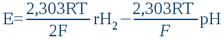(13)

The resulting Equation (13) is of paramount importance because it shows ORP’s second ambiguity . The redox potential E (also known as ORP) is the algebraic sum of two terms: the first term on the right side of Equation (13) depends only on the electron exchanges rH2, the second only on proton exchanges pH. What we therefore measure in a solution using a voltmeter depends on both pH and rH2. To rule on the oxidative or the reductive (i.e. antioxidative) character of a solution, solely based on an ORP measurement and regardless of the solution’s pH leads to miscalculations in the overwhelming majority of cases.


Therefore knowing the physical meaning of Equation (13), to assert – as has been done by eminent colleagues – that « the rH2 does not exist because it is the arbitrary linear combination of two experimental measurements, the potential E (or ORP) and the pH » is strange to say the least. It is all the more strange when you consider that experimental measurements of pH and rH2 relate both values to a measurement of a battery’s electromotive force (emf). According to their logic, one could also argue that the pH does not exist because it is the linear combination of two other experimental measurements: the ORP and rH2.

#### Practical formula for calculating rH2

Given the fact that at a temperature of 25°C = 298K, the numerical value of the term 2F/2.303RT = 33.8, from Equation (13) it is easy to explain rH2:

rH2 = 33.8 E + 2 pH
(14)

In this equation, E is the (corrected) ORP expressed in volts.

Important Note: To properly apply Equation (14), you must take into account that the true ORP is not the value indicated by the voltmeter connected to an ORP electrode (i.e. the platinum electrode)! We must insist: the instrument does not measure the ORP, but the difference in potential between a platinum electrode immersed in the solution and the reference silver chloride electrode whose potential, compared to the standard hydrogen electrode, is +200 mV at 25°C. So to get the correct ORP value (designated E in equation 14) with readings on the instrument, you must add 200 mV. Careful: in the calculations, E must always be expressed in Volts (n.b. 1 Volt = 1000 mV).

For example, calculate the rH2 of « Dasani » water whose pH is 3.04 and the potential measured by the voltmeter is +290 mV. The value of the ORP (or E) is obtained by adding the Ag/AgCl reference electrode potential’s 200 mV to the measured value +290 mV: therefore E=290+200=490mV = 0.49 Volt; pH = 3.04. Introduce these values in Equation (14), to obtain:

rH2 = 33.8 x 0.49 + 2 x 3.04 = 22.6

Despite its clearly acidic character, this water naturally has a reductive character (i.e. antioxidative).

Now consider a clearly alkaline water, « Essentia » water having a pH = 9.26 and a measured ORP of +179 mV = 0.179 Volt. Its rH2 = 33.8 x (0.179 + 0.200) + 2 x 9.26 = 31.3. This water, despite its alkaline nature and its lower ORP (compared to Dasani water) is in fact oxidative.

By basing one’s decision solely on the values displayed by the voltmeter, one would have said that « Dasani water (+290 mV) is more oxidative than Essentia water (+179 mV) », while the opposite is true.

## Vincent’s Quantization Value (W)

In medical bioelectronics, we often use a concept established in 1973 by Louis-Claude Vincent. Starting from bioelectronic coordinates, Vincent developed a methodology for assessing the « level of energy stored in the body » and that energy which is « eliminated ». To this end, he applied the laws of conventional electricity to redox ORP (which we refer to in the calculations as E) and the aqueous medium’s resistivity ρ (pronounced as « rho »).

It’s an empirical notion that even Vincent did not see fit to give the precise physical meaning for. In 1992, we attempted to establish a thermodynamic model for this notion. [Ref.: Országh J., Some physicochemical aspects of bio-electronic coordinates, Sciences du Vivant (Éd. Arys, Paris), volume 4, pages 45-62 (1992)]

All redox systems can be diagrammed with a battery consisting of a standard hydrogen electrode and an electrode in solution containing the redox system studied. This battery’s electromotive force (or Gibbs to a constant) is obviously equal to E. By convention , (and as assumed implicitly by L.-C. Vincent), we can assume that an inert electrode having a surface area s = 1 cm2 is immersed in a proportion of 1 cm3 of this electrolyte whose resistivity is ρ. The maximum power W of this battery will thus be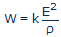(15)

...where k is a constant whose numerical value is 1 cm2/cm. If E is expressed in millivolts and ρ in ohm.cm, we get W in microwatts (µW). Actually, this constant is the ratio of the electrode’s surface s to the distance ℓ traveled by the current through the battery: k=s/ℓ. The W term expresses the maximum speed of electric energy production in this hypothetical and conventional battery.


Although not explicitly mentioned in the works of Vincent, this convention is implicitly present. If the constant k was different from 1, Vincent’s formulae would not give the values calculated by their author. In fact, Vincent never indicated k in his formulae, which therefore are inconsistent from a dimensional perspective. It’s to resolve this inconsistency and give it a physical meaning that we introduced k .

Now place the E value derived from Equation (14) into Equation (15), taking into account that k=1cm²/cm: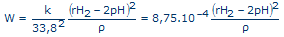(16)

The 8.75.10–4 value is only valid at 25° C. At a different temperature T, you need to replace the 33.8 constant by 2F/2.303RT.

In practice, measuring instruments don’t display the resistivity ρ, but rather its reciprocal value, conductivity κ (pronounced « kappa »): κ=1/ρ. If the conductivity is expressed in µS/cm (pronounced « microSiemens per cm »), the practical formula for calculating W is:

W = 8.75.10–4 κ (rH2 – 2pH)2
(17)

In this case, you obtain Vincent's Quantization value W, in µW (pronounced « microwatt »).

For example, calculate the Vincent quantization W of city tap water whose conductivity is κ = 650 µS/cm, with an rH2 = 30.5 and pH = 7.6:

W = 8.75.10–4 x 650 x (30,5 – 2x7.6)2 = 133 µW

In comparison, biocompatible water obtained by rainwater microfiltration has a conductivity κ = 35 µS/cm, rH2 = 28.5 et pH= 7.2:

W = 8.75.10–4 x 35 x (28.5 – 2x7.5)2 = 5.6 µW

TOP